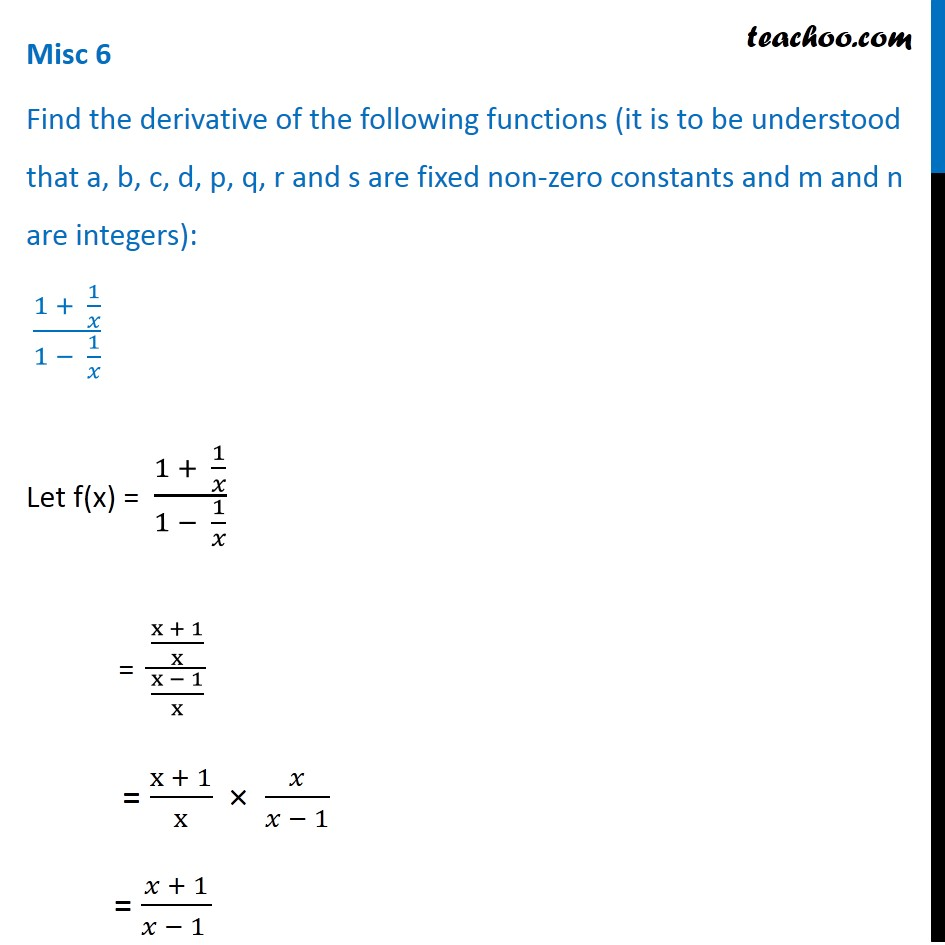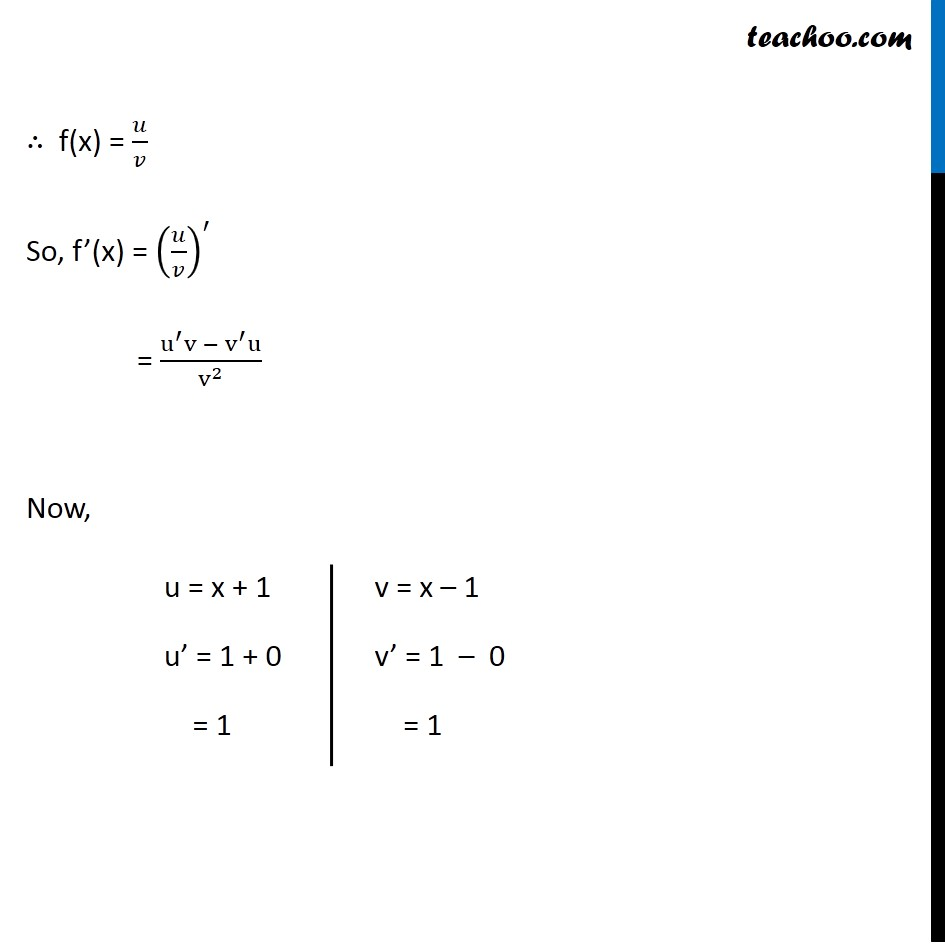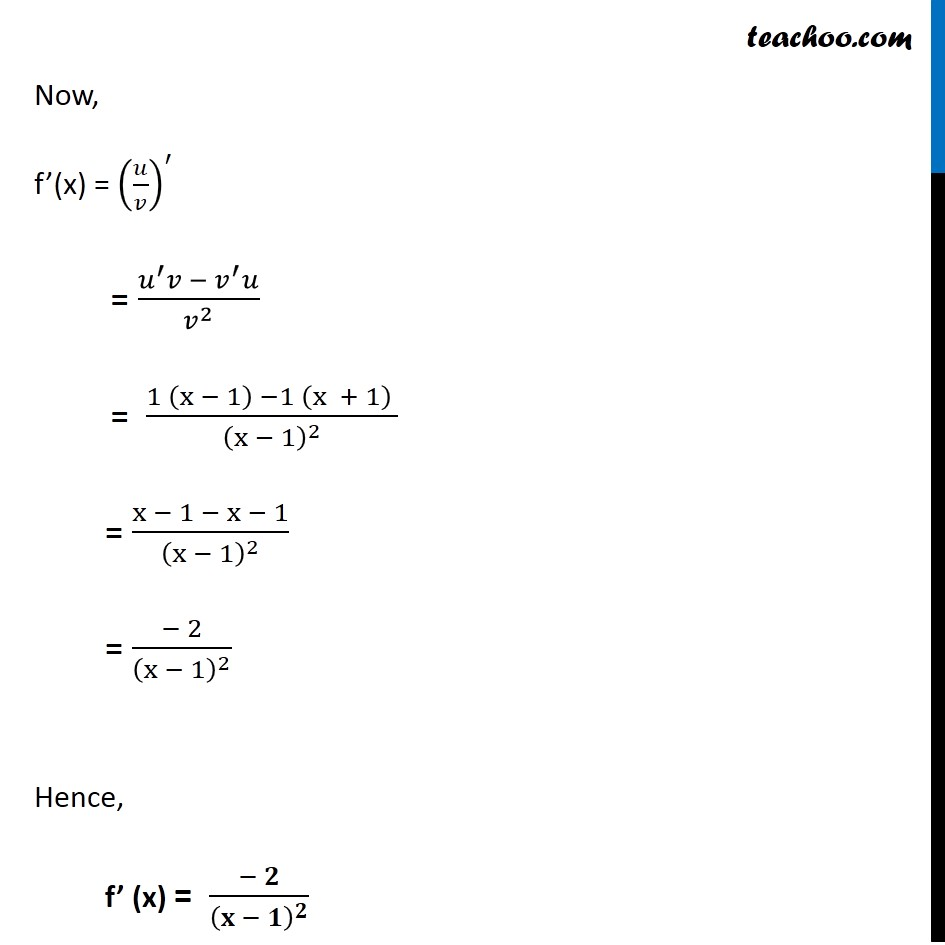Miscellaneous

Chapter 12 Class 11 Limits and Derivatives
Serial order wiseLearn in your speed, with individual attention - Teachoo Maths 1-on-1 Class

### Transcript

Misc 6 Find the derivative of the following functions (it is to be understood that a, b, c, d, p, q, r and s are fixed non-zero constants and m and n are integers): (1 + 1/𝑥)/(1 − 1/𝑥) Let f(x) = (1 + 1/𝑥)/(1 − 1/𝑥) = ((x + 1)/x)/((x − 1)/x) = (x + 1)/x × 𝑥/(𝑥 − 1) = (𝑥 + 1)/(𝑥 − 1 ) So, f(x) = (𝑥 + 1)/(𝑥 − 1 ) ∴ f(x) = 𝑢/𝑣 So, f’(x) = (𝑢/𝑣)^′ = (u^′ v − v^′ u)/v^2 Now, u = x + 1 u’ = 1 + 0 = 1 v = x – 1 v’ = 1 – 0 = 1 Now, f’(x) = (𝑢/𝑣)^′ = (𝑢^′ 𝑣 −〖 𝑣〗^′ 𝑢)/𝑣^2 = (1 (x − 1) −1 (x + 1) )/(x − 1)^2 = (x − 1 − x − 1)/(x − 1)^2 = (− 2)/(x − 1)^2 Hence, f’ (x) = (− 𝟐)/(𝐱 − 𝟏)^𝟐